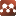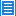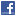## Geometry## Remarks on Liouville-Type Theorems on Complete Noncompact Finsler Manifolds

Authors: Songting Yin, Pan Zhang

In this paper, we give a gradient estimate of positive solution to the equation $$\Delta u=-\lambda^2u, \ \ \lambda\geq 0$$ on a complete non-compact Finsler manifold. Then we obtain the corresponding Liouville-type theorem and Harnack inequality for the solution. Moreover, on a complete non-compact Finsler manifold we also prove a Liouville-type theorem for a $C^2$-nonegative function $f$ satisfying $$\Delta f\geq cf^d, c>0, d>1,$$ which improves a result obtained by Yin and He.